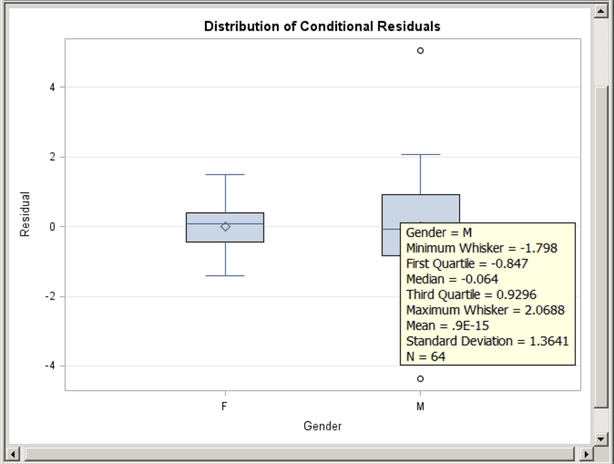## Example 21.1 Creating Graphs with Tool Tips in HTML

This example demonstrates how to request graphs in HTML that are enhanced with tooltip displays, which appear when you move a mouse over certain features of the graph. When you specify the HTML destination and the IMAGEMAP=ON option in the ODS GRAPHICS statement, an image map of coordinates for tooltips is generated along with the HTML output file. Individual graphs are saved as PNG files.

Example 58.2 and Example 58.8 of Chapter 58, The MIXED Procedure, analyze a data set with repeated growth measurements for 27 children. The following step creates the data set:

```data pr;
input Person Gender \$ y1 y2 y3 y4 @@;
y=y1; Age=8;  output;
y=y2; Age=10; output;
y=y3; Age=12; output;
y=y4; Age=14; output;
drop y1-y4;
datalines;
1  F  21.0  20.0  21.5  23.0      2  F  21.0  21.5  24.0  25.5

... more lines ...

;
```

The following statements fit a mixed model with random intercepts and slopes for each child:

```ods _all_ close;
ods graphics on / imagemap=on;
ods html body='b.html' style=HTMLBlue;

proc mixed data=pr method=ml plots=boxplot;
ods select 'Conditional Residuals by Gender';
class Person Gender;
model y = Gender Age Gender*Age;
random intercept Age / type=un subject=Person;
run;

ods html close;
```

The PLOTS=BOXPLOT option in the PROC MIXED statement requests box plots of observed values and residuals for each classification main effect in the model (Gender and Person). Only the by-gender box plots are actually created due to the ODS SELECT statement, which uses the plot label to select the plot. Output 21.1.1 displays the results. Moving the mouse over a box plot displays a tooltip with summary statistics for the class level. Graphics with tooltips are supported for only the HTML destination.

Output 21.1.1 Box Plot with Tool Tips